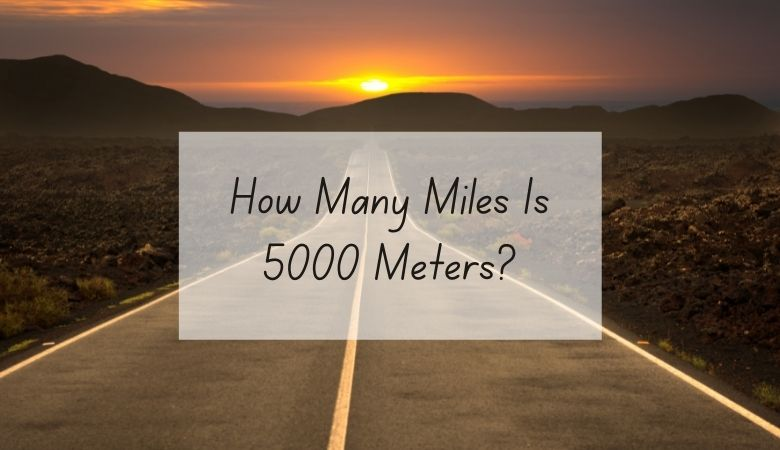# How Many Miles Is 5000 Meters?

There are 3.10752 miles in a 5000 meters.

In order to find out how many miles are in a certain number of meters, you would need to divide the number of meters by 1,609.

For example, if you want to know how many miles are in 5000 meters, divide 5000 by 1,609.

The answer, which is 3.10752, tells you that there are that many miles in 5000 meters.

If you want to reverse the question and figure out how many meters can be made from a certain number of miles, you would multiply the number of miles by 1,609.

For example, if you have 5 miles, you can multiply it by 1,609 to get 8045.

This means that there are 8045 meters in 5 miles.

If you don’t feel like doing the math, use the meters into miles conversion calculator below.

Also, check out our online conversion calculator to convert different units of measurements and quickly give you the answer.

You can also use the following table to convert meters into miles.

## Meters And Miles Measurements

Meters are part of the metric system of measurement, which is common around the world thanks to its ease of use.

Although the metric system isn’t standard in the United States, it’s still commonly used for scientific measurements.

Miles are part of the imperial system of measurement, which is standard in the United States.